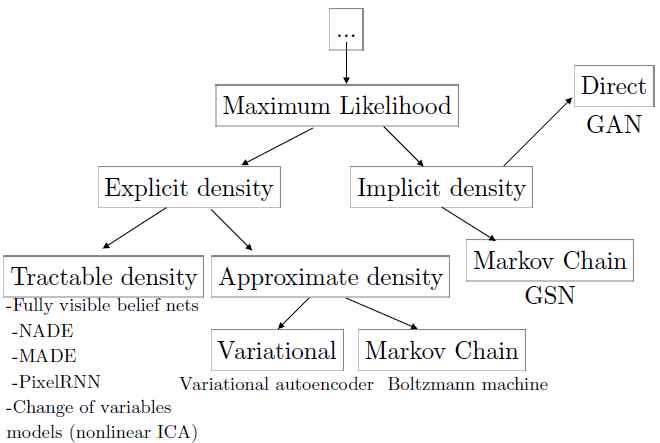1. GAN的提出和其他生成式模型的比较
2. 经典GAN的变换成JS散度的理论推导。
3. 经典GAN由于散度的度量导致的训练不稳定和mode collapse背后的数学解释。

## 1. brief of GAN GAN的简介

1. 李宏毅老师的课程。对抗生成网络(GAN)国语教程(2018)
2. Ian Goodfellow, NIPS 2016的slides2016-12-04-NIPS
3. 沈鹏的翻译和整理 GAN介绍

### 1.2 why generative model

1. 应用到强化学习中：
• 将产生式模型用于对假设环境的增强学习， 这样即使发生错误行为也不会造成实际的损失。 通过跟踪以前不同状态被访问的频率，或者不同的行为被尝试的频率，生成式模型也可以用于指导探索者/探险者。 生成式模型，特别是GAN，也可以用于inverse reinforcement learning。
2. 可以使用有缺失的数据训练和预测
3. 可以应对多任务学习
4. 很多任务本身需要根据分布来产生真实数据。比如：低分辨率产生超高分辨率数据；艺术创作任务（随意画线产生图片）。

### 1.3 简单理解GAN的算法过程

（这里的公式和原论文的会有区别，后面就讲到发生了什么。）

• 本质上，Generator的自我训练，也可以使用监督学习的方式。但是，可能并没有那么多的训练数据对。

• 而Discriminator也可以用于生成图片：在穷举所有可能的x，只需要得到最大的D(xi)，而xi便是生成的图片。但是求解argmax的计算量很难（线性方程还是可以穷举的）。

## 2. GAN ： structured learning• one-shot/ zero-shot learning：可以认为是极端的小样本学习
• 要求算法有大菊观，能把各部件很好地组合

GAN可以认为是解决Structured Learning的一种解决方案。传统的解决方案有两类：

1. Bottom-up。相当于生成多个component，独立生成。（Generator）
2. Top-Down。相当于从上帝视角评估整个方案，选择最好的组合，可以抓住多个component的相关关系。（Discriminator）

• VAE对超参数不敏感，产生的数值会比较稳定
• GANs对超参数敏感，产生数据的上下限变化较大
• VAE使用MSE评估错误（即优化MSE），这个模型被强制为只能选择下一帧多个答案中的一个正确答案。 因为下一帧图像有很多的可能性， 这些可能的答案会有些细微的位置上的差别， 单一的答案选择让模型针对这些细微差别做了平均化处理，可能会使得生成的matrix糢糊。有很多任务，一个输入可能对应多个正确的输出， 每一个输出都是可接受的。 传统的基于平均值的机器学习模型， 比如， 对期望输出和预测输出的均方差（MSE）进行最小化的方法，无法训练此种有多个正确输出的模型。GAN能应对这种问题

## 3. Theory behind GAN

### 3.1. Maximum Likelihood Estimation

S1: 抽样m个样本：$\left{x^1, x^2, \ldots, x^m\right}$ ， 计算 $P_G(x^i;\theta)$， 即被G的分布抽样出来的概率。

S2: 最大似然：$L=\prod_{i=1}^m P_G\left(x^i ; \theta\right)$，即最小似然的负对数。或者最小化KL散度（KL Divergence）（生成模型预设的分布与实际分布的KL散度）

S3：更新$\theta$.

$E_{x \sim P_{\text {data}}}$指从Pdata中抽样x。### 3.2. Generator

1. 正态分布抽样（或者其他抽样）生成z
2. 使用NN的生成器G，得到$x = G(z)$ ，通过神经网络，就得到了复杂的分布$P_G$。
3. 目标：最小化$P_G$ 和实际分布的差异$G^*=\arg \min_G Div\left(P_G, P_{\text {data}}\right)$

### 3.3. Discriminator

#### 3.3.2 小结

1. 如果两个分布之间没有重叠，或者部份重叠时，JS散度为多少呢？

#### 3.3.3 Why minimax??

1. max what : 在训练的第一步，我们需要提高判别器的辨别能力。即在给定生成器$G_i$的情况下，找到D的参数$\theta_d$最大化判别器对不同分布样本的JS散度（区分能力）。
2. min what： 与此同时，对于当前分辩能力最好的判别器$D_i$，找到$\theta_g$，使得判别器分辩两类样本的区分度最小。

#### 3.3.4 散度的选择

KL散度不是对称的； 最小化$D_{K L}\left(p_{\text {model}}| | p_{\text {data}}\right)$与最小化$D_{K L}\left(p_{d a t a} | p_{m o d e l}\right)$是不同的。### 3.4. 更新过程1. 初始化G $\theta _g$，D $\theta_d$
2. 训练判别器，$\max_D V(G,D)$
1. 分布在$P_{data}$和$P_G$中，抽样m个数据。（$P_G$的抽样值，随机m个向量z，使得$\widetilde{x} = G(z)$），最大化目标函数

​ 固定G，训练D：$P_{data}$的数据为正样本，$P_G$为负样本，最小化交叉熵，训练“是否是生成样本”的二分类器

​ 更新D的参数：

1. 以上，变获得了JS散度的度量方式：判别器D。这边，可以训练多次D。

2. 训练生成器，减少JS散度的绝对值大小$\min_G \max_D V(G, D)$

1. 抽样m个$P_G$.

2. 最小化目标函数

3. 更新权重

4. 注意：为了避免D和G的变换导致G的可行解范围发生很大变换，在训练过程中，应该让G的更新幅度要小。即更新一次就好。

3. 重复K次 2~4，

#### 3.4.1 小结：梯度不稳定和mode collapse/mode dropping

• 第一是它同时要最小化生成分布与真实分布的KL散度，却又要最大化两者的JS散度，在数值上会导致梯度不稳定。
• 第二是，KL散度是不对称的，当某一项P(x)趋近于0，另一项为1时，KL散度会是0或者正无穷。

1. 当$P_g(x) \rightarrow 0$而$P_d(x) \rightarrow 1$时，$P_g(x) \log \frac{P_g(x)}{P_d(x)} \rightarrow 0$, 对$K L\left(P_g | P_d\right)$贡献趋近0.
2. 当$P_g(x) \rightarrow 1$而$P_d(x) \rightarrow 0$时，$P_g(x) \log \frac{P_g(x)}{P_d(x)} \rightarrow+\infty$, 对$K L\left(P_g | P_d\right)$贡献趋近正无穷 。

## 4. GAN与其他生成模型的比较

• 它可以并行产生样本， 而不是使用运行时间与xx的维数成比例的方法。 这一点比FVBN有优势。
• 生成函数设计有很少的限制。 这一点是针对玻尔兹曼机的优势， 只有少数的概率分布能够被马尔可夫链来处理， 并且相比非线性ICA， 生成器必须是可逆的，并且隐变量编码z必须与样本x同维。
• 不需要马尔可夫链。 这一点比玻尔兹曼机和生成随机网络有优势。
• 不需要变分边界， 并且那些被用于GAN的模型是全局优化器， 所以GAN是渐近一致的（asymptotically consistent）。 一些VAE也可能是渐近一致，但是还没有被证明。
• GAN通常被认为比其他方法可以产生更好的样本。

0%